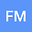Saturation Point and Phase Envelope Calculation for Reactive Systems Based on the RAND Formulation
•••• Fernando de Azevedo Medeiros,
• Erling Halfdan Stenby,
• Wei Yan
Fernando de Azevedo Medeiros

Corresponding Author:[email protected]

Author ProfileErling Halfdan Stenby
Center for Energy and Resources Engineering, Department of Chemistry, Technical University of Denmark
Wei Yan
Center for Energy and Resources Engineering, Department of Chemistry, Technical University of Denmark

## Abstract

Analysis of multicomponent reactive systems requires reliable and accurate equilibrium calculation. There are many stoichiometric or non-stoichiometric methods to solve the flash-type calculations of a mixture in chemical and phase equilibrium. In contrast, there is a lack of robust and efficient methods for another important type of equilibrium calculation, the saturation point calculation or the calculation under the phase fraction specification (β-specification), for a reactive mixture. In this work, we developed RAND-based algorithms for calculating the saturation points and phase envelope of a reactive mixture. The RAND formulation is a non-stoichiometric approach recently extended to non-ideal mixtures for different flash specifications. We showed here how to modify the RAND-based flash formulation to solve the β-specification problems. We distinguished between two types of phase fractions, the one based on components and the one based on elements. They led to different constraint equations in the formulation. Furthermore, we introduced element-based partition coefficients, similar to the equilibrium ratios or K-factors used for non-reactive mixtures. Use of these new variables is essential to cross the critical point of a reactive mixture in the phase envelope construction. Since the formulation developed for reactive mixtures is general, it can also be reduced and used for the simpler non-reactive mixtures. We showed how the reduction could be made and how the reduced algorithm served as an alternative approach to the prevailing phase envelope algorithm of Michelsen. We illustrated the robustness and efficiency of the proposed algorithm using four examples: Pxy diagrams for CO2-NaCl brine, a solid-liquid T xy diagram for MgCl2-water, a PT phase envelope for a reactive mixture with the alkene hydration reaction, and a PT phase envelope for a non-reactive hydrocarbon mixture.